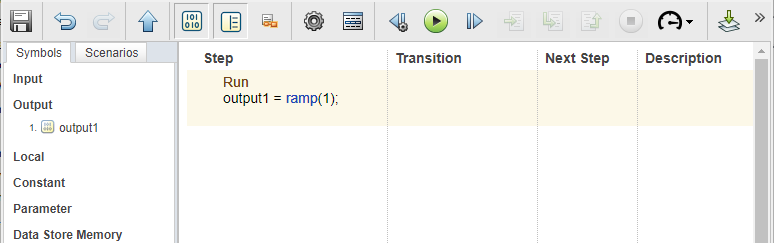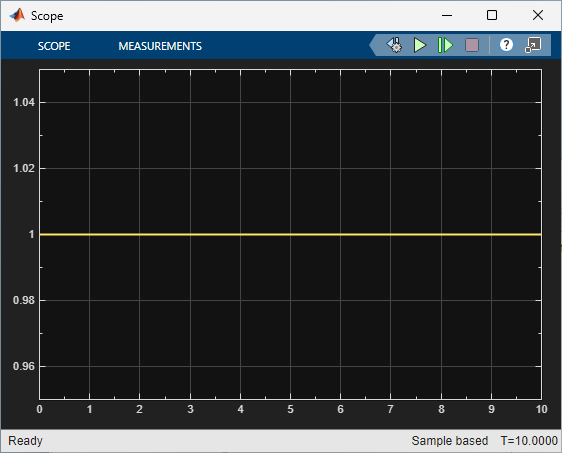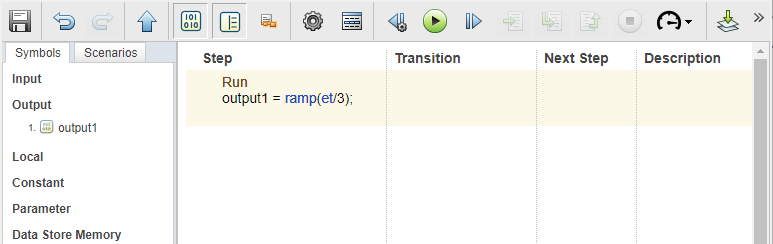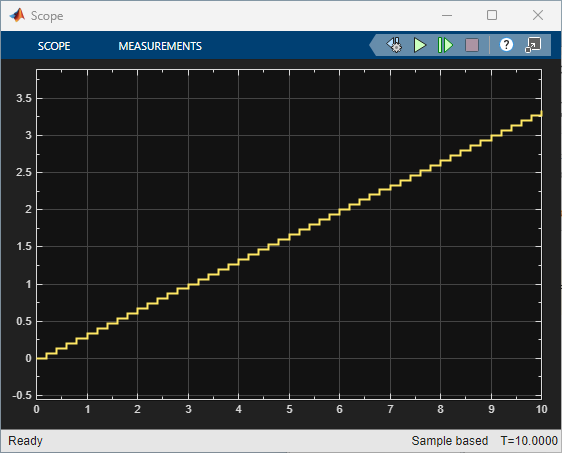# ramp

Return input value for test signal generation

## Syntax

``y = ramp(x)``

## Description

example

````y = ramp(x)` returns the value of `x`. Use the `ramp` operator in the test step of a Test Sequence or Test Assessment block.```

## Examples

expand all

In a Test Sequence block, output a constant signal of value `1` while the test step is active.The Test Sequence block outputs a signal equal to `1`:In a Test Sequence block, generate a signal that increases one unit every 3 seconds in the test step.The Test Sequence block outputs a signal equal to `et/3`:## Input Arguments

expand all

Value during the test step, specified as a numeric scalar.

Data Types: `single` | `double` | `int8` | `int16` | `int32` | `int64` | `uint8` | `uint16` | `uint32` | `uint64`

## Version History

Introduced in R2015a